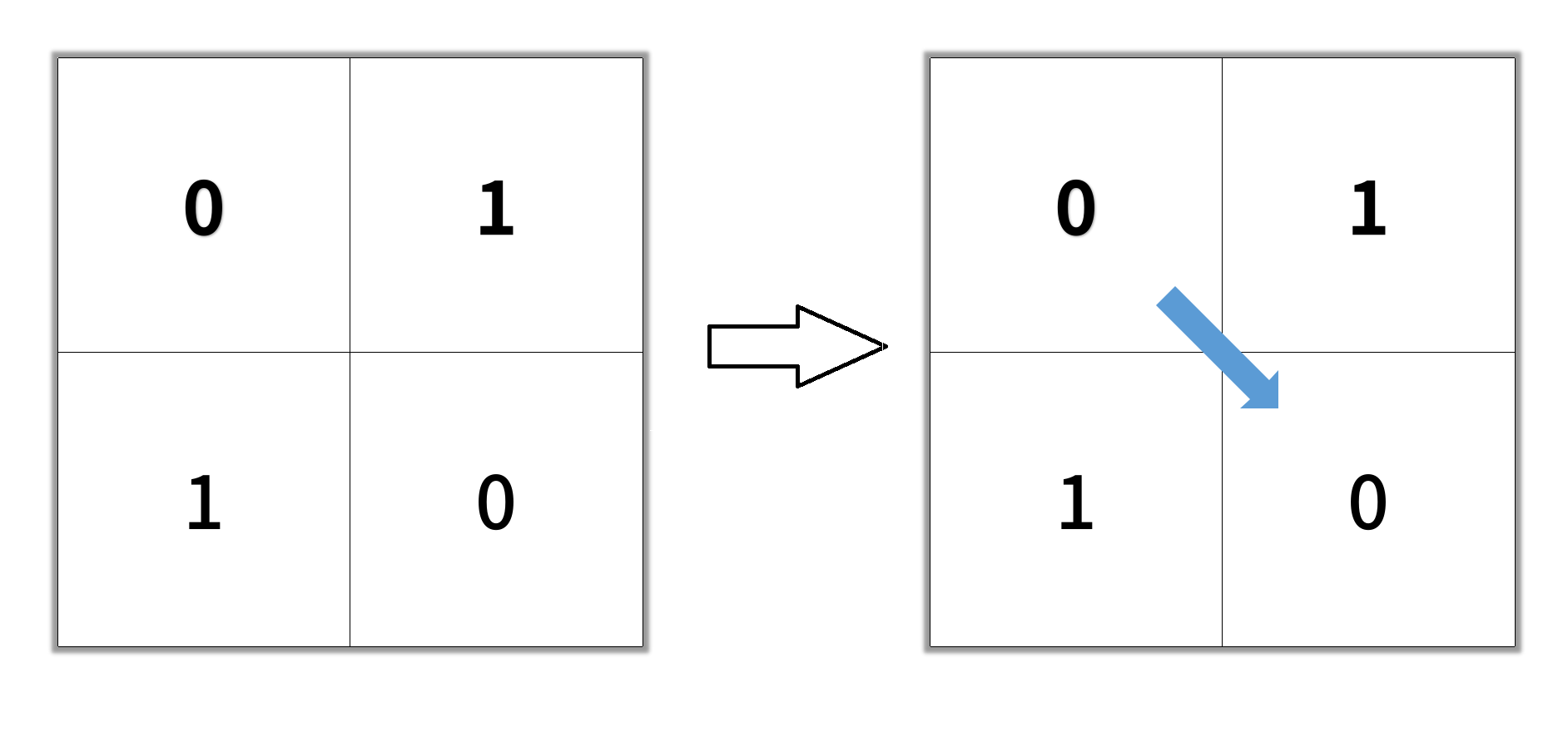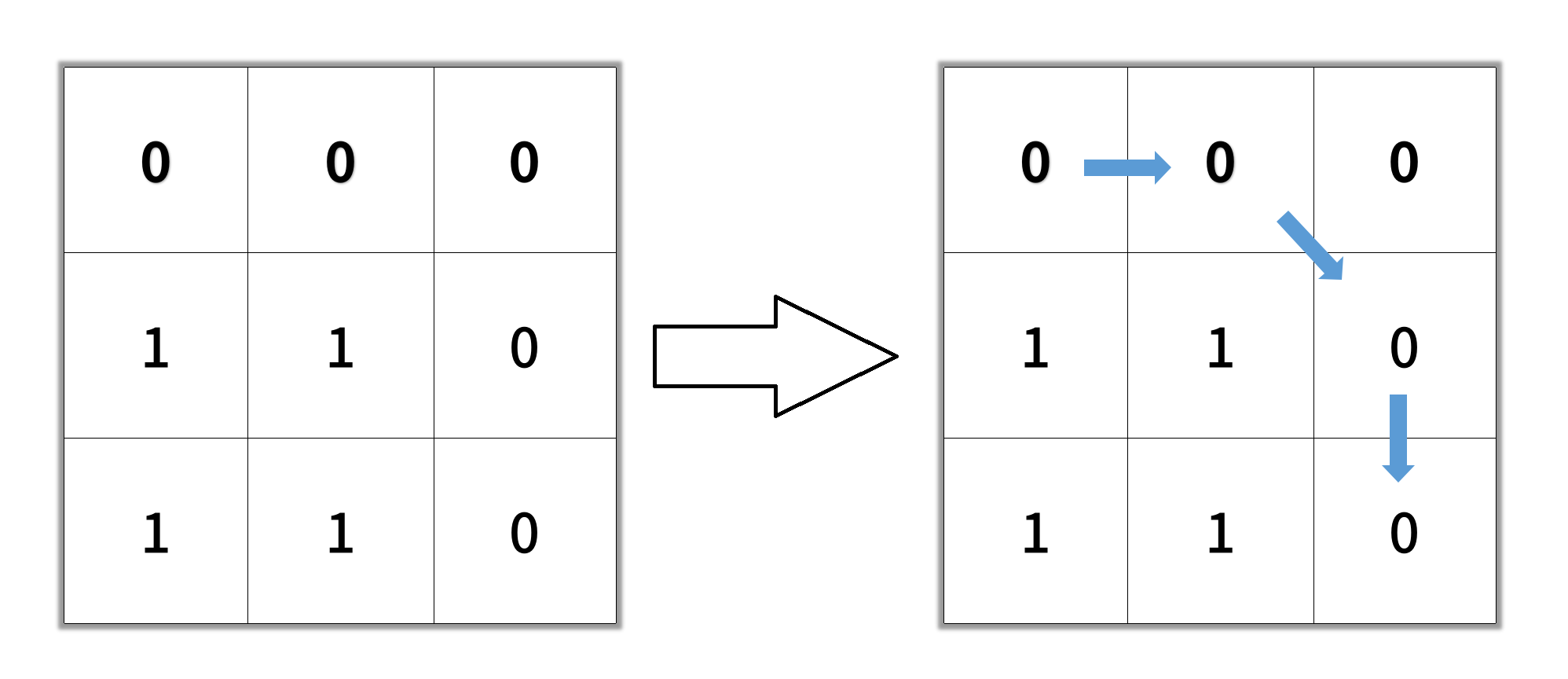# GeetCode Hub

Given an `n x n` binary matrix `grid`, return the length of the shortest clear path in the matrix. If there is no clear path, return `-1`.

A clear path in a binary matrix is a path from the top-left cell (i.e., `(0, 0)`) to the bottom-right cell (i.e., `(n - 1, n - 1)`) such that:

• All the visited cells of the path are `0`.
• All the adjacent cells of the path are 8-directionally connected (i.e., they are different and they share an edge or a corner).

The length of a clear path is the number of visited cells of this path.

Example 1:```Input: grid = [[0,1],[1,0]]
Output: 2
```

Example 2:```Input: grid = [[0,0,0],[1,1,0],[1,1,0]]
Output: 4
```

Example 3:

```Input: grid = [[1,0,0],[1,1,0],[1,1,0]]
Output: -1
```

Constraints:

• `n == grid.length`
• `n == grid[i].length`
• `1 <= n <= 100`
• `grid[i][j] is 0 or 1`

class Solution { public int shortestPathBinaryMatrix(int[][] grid) { } }